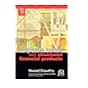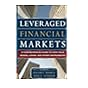Normal view

Material type:TextPublisher: San Diego, CA : Butterworth-Heinemann, 2015Description: 1 online resource : illustrationsContent type: text Media type: computer Carrier type: online resourceSubject(s): Bonds | Investment analysisDDC classification: 332.63234 Online resources: eBook-ScienceDirect
Contents:
""Front Cover""; ""Advanced Fixed Income Analysis""; ""Copyright""; ""Dedication""; ""Contents""; ""About the Authors""; ""Preface""; ""Preface to the First Edition (published 2004)""; ""The dynamics of the yield curve""; ""Factors influencing the yield curve""; ""Approaches to modelling""; ""One-factor, two-factor and multi-factor models""; ""The short-term rate and the yield curve""; ""Arbitrage-free and equilibrium modelling""; ""Risk-neutral probabilities""; ""Mathematics primer""; ""Random variables and probability distributions""; ""Continuous random variables""; ""Expected values""
""Regression analysis""""Stochastic processes""; ""Stochastic calculus""; ""Selected Bibliography""; ""Chapter 1: Asset-Swap Spreads and Relative Value Analysis""; ""1.1. Asset-Swap Spread""; ""1.2. Swap Spread for Richness and Cheapness Analysis""; ""1.3. Z-Spread Measure""; ""1.4. The Credit Default Swap Basis and Trading Issues""; ""1.5. Analysis Using Market Observation""; ""Appendix1. The Par Asset-Swap Spread""; ""Bibliography""; ""Chapter 2: The Dynamics of Asset Prices""; ""2.1. The Behaviour of Asset Prices""; ""2.1.1. Stochastic Processes""
""2.1.2. Wiener Process or Brownian Motion""""2.1.3. The Martingale Property""; ""2.1.4. Generalised Wiener Process""; ""2.1.5. A Model of the Dynamics of Asset Prices""; ""2.1.6. The Distribution of the Risk-Free Interest Rate""; ""2.2. Stochastic Calculus Models: Brownian Motion and It�A? Calculus""; ""2.2.1. Brownian Motion""; ""2.2.2. Stochastic Calculus""; ""2.2.3. Stochastic Integrals""; ""2.2.4. Generalised It�A?s Formula""; ""2.2.5. Information Structures""; ""2.3. Perfect Capital Markets""; ""2.3.1. Stochastic Price Processes""; ""2.3.2. Perfect Markets""
""2.3.3. Uncertainty of Interest Rates""""2.3.4. Asset Price Processes""; ""Appendix A. An Introduction to Stochastic Processes""; ""Appendix B. It�A?s Lemma""; ""Appendix C. Derivation of It�A?s Formula""; ""Appendix D. The Integral""; ""Selected Bibliography and References""; ""Chapter 3: Interest-Rate Models I""; ""3.1. Introduction""; ""3.1.1. Bond Price and Yield""; ""3.1.2. Interest-Rate Models""; ""3.1.3. Introduction to Bond Analysis Using Spot Rates and Forward Rates in Continuous Time""; ""3.1.3.1. The Spot and Forward Rate Relationship""
""3.1.3.2. Bond Prices as a Function of Spot and Forward Rates""""3.2. Interest-Rate Processes""; ""3.3. One-Factor Models""; ""3.3.1. The Vasicek Model""; ""3.3.2. The Merton Model""; ""3.3.3. The Cox-Ingersoll-Ross Model""; ""3.3.4. General Comment""; ""3.4. Arbitrage-Free Models""; ""3.4.1. The Ho and Lee Model""; ""3.4.2. The Hull-White Model""; ""3.4.3. The Black-Derman-Toy Model""; ""3.5. Fitting the Model""; ""3.6. Summary""; ""3.7. Website Models""; ""Appendix. Illustration of Forward Rate Structure When Spot Rate Structure Is Increasing""; ""Selected Bibliography and References""
Summary: Each new chapter of the Second Edition covers an aspect of the fixed income market that has become relevant to investors but is not covered at an advanced level in existing textbooks. This is material that is pertinent to the investment decisions but is not freely available to those not originating the products. Professor Choudhry's method is to place ideas into contexts in order to keep them from becoming too theoretical. While the level of mathematical sophistication is both high and specialized, he includes a brief introduction to the key mathematical concepts. This is a book on the finan.
List(s) this item appears in: ทรัพยากรใหม่ประจำเดือน มกราคม 2564
Tags from this library: No tags from this library for this title.Average rating: 0.0 (0 votes)
Item type Home library Collection Call number Status Date due Barcode Item holdsE-book UTCC Library
E-Books (English) 332.63234 C552a (Browse shelf) Online Access
Total holds: 0
##### Browsing UTCC Library shelves Close shelf browserNo cover image available332.6323 ส558ก การลงทุนในตราสารหนี้ / 332.6323 ส558ก การลงทุนในตราสารหนี้ / 332.63234 C552a Advanced fixed income analysis / 332.63234 C552a Advanced fixed income analysis / 332.63234 C552c Corporate bonds and structured financial products / 332.63234 L659 Leveraged financial markets : 332.6324 ค86ฟ ฟองสบู่ (แตก?) :

""Front Cover""; ""Advanced Fixed Income Analysis""; ""Copyright""; ""Dedication""; ""Contents""; ""About the Authors""; ""Preface""; ""Preface to the First Edition (published 2004)""; ""The dynamics of the yield curve""; ""Factors influencing the yield curve""; ""Approaches to modelling""; ""One-factor, two-factor and multi-factor models""; ""The short-term rate and the yield curve""; ""Arbitrage-free and equilibrium modelling""; ""Risk-neutral probabilities""; ""Mathematics primer""; ""Random variables and probability distributions""; ""Continuous random variables""; ""Expected values""

""Regression analysis""""Stochastic processes""; ""Stochastic calculus""; ""Selected Bibliography""; ""Chapter 1: Asset-Swap Spreads and Relative Value Analysis""; ""1.1. Asset-Swap Spread""; ""1.2. Swap Spread for Richness and Cheapness Analysis""; ""1.3. Z-Spread Measure""; ""1.4. The Credit Default Swap Basis and Trading Issues""; ""1.5. Analysis Using Market Observation""; ""Appendix1. The Par Asset-Swap Spread""; ""Bibliography""; ""Chapter 2: The Dynamics of Asset Prices""; ""2.1. The Behaviour of Asset Prices""; ""2.1.1. Stochastic Processes""

""2.1.2. Wiener Process or Brownian Motion""""2.1.3. The Martingale Property""; ""2.1.4. Generalised Wiener Process""; ""2.1.5. A Model of the Dynamics of Asset Prices""; ""2.1.6. The Distribution of the Risk-Free Interest Rate""; ""2.2. Stochastic Calculus Models: Brownian Motion and It�A? Calculus""; ""2.2.1. Brownian Motion""; ""2.2.2. Stochastic Calculus""; ""2.2.3. Stochastic Integrals""; ""2.2.4. Generalised It�A?s Formula""; ""2.2.5. Information Structures""; ""2.3. Perfect Capital Markets""; ""2.3.1. Stochastic Price Processes""; ""2.3.2. Perfect Markets""

""2.3.3. Uncertainty of Interest Rates""""2.3.4. Asset Price Processes""; ""Appendix A. An Introduction to Stochastic Processes""; ""Appendix B. It�A?s Lemma""; ""Appendix C. Derivation of It�A?s Formula""; ""Appendix D. The Integral""; ""Selected Bibliography and References""; ""Chapter 3: Interest-Rate Models I""; ""3.1. Introduction""; ""3.1.1. Bond Price and Yield""; ""3.1.2. Interest-Rate Models""; ""3.1.3. Introduction to Bond Analysis Using Spot Rates and Forward Rates in Continuous Time""; ""3.1.3.1. The Spot and Forward Rate Relationship""

""3.1.3.2. Bond Prices as a Function of Spot and Forward Rates""""3.2. Interest-Rate Processes""; ""3.3. One-Factor Models""; ""3.3.1. The Vasicek Model""; ""3.3.2. The Merton Model""; ""3.3.3. The Cox-Ingersoll-Ross Model""; ""3.3.4. General Comment""; ""3.4. Arbitrage-Free Models""; ""3.4.1. The Ho and Lee Model""; ""3.4.2. The Hull-White Model""; ""3.4.3. The Black-Derman-Toy Model""; ""3.5. Fitting the Model""; ""3.6. Summary""; ""3.7. Website Models""; ""Appendix. Illustration of Forward Rate Structure When Spot Rate Structure Is Increasing""; ""Selected Bibliography and References""

Each new chapter of the Second Edition covers an aspect of the fixed income market that has become relevant to investors but is not covered at an advanced level in existing textbooks. This is material that is pertinent to the investment decisions but is not freely available to those not originating the products. Professor Choudhry's method is to place ideas into contexts in order to keep them from becoming too theoretical. While the level of mathematical sophistication is both high and specialized, he includes a brief introduction to the key mathematical concepts. This is a book on the finan.

There are no comments on this title.